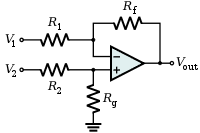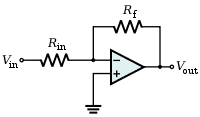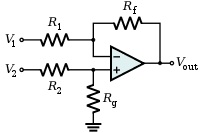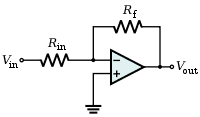# Differentiating Op-Amp basic exercise

Gold Member

## Homework Statement

Given here is an Operational Amplifier, its feeding Voltages are +-10.

A) Calculate Vin to get a Vout of -2V, point out the direction of the current in this case (From a to b or b to a)

http://img72.imageshack.us/img72/2550/needheed.jpg [Broken]

## The Attempt at a Solution

http://img844.imageshack.us/img844/4493/blury.jpg [Broken]

Is this the right formula?

The Vx's are at point a and c, that I know.

Last edited by a moderator:

I like Serena
Homework Helper
How did you get this formula?
And what is V1?

Do you have a list of relevant equations?

Last edited:
This is an amplifier with negative feedback. The main things you need to know about such an amplifier are:
1) The V at the - and the V at the + inputs can be assumed to be equal (V at a = V at c = +4V)
2) the input resistance is infinite, this means that no current flows into or out of the - and + input terminals.
the thing to do now is calculate some currents.
Current will flow from V in through R1 towards the - input, so Iin = V/R1, V is the voltage ACROSS R1
Iin = (Vin- 4)/R1....... check that you are OK with this !!!
This current does NOT flow into the -input, so it must go up through R2.
This current passing through R2 will produce a PD = I x R2
So a voltage of R2 x (Vin-4)/R1.....( 2Vin -8) ...... put in the values of R1 and R2) will be developed across R2 (this is the familiar form of the equation for the gain of an amplifier of this sort.... I hope you recognise it!!)
I have the current flowing from left to right through R1 and R2 so this means that the voltage at the right hand end of R2 must be the voltage at the - terminal MINUS the voltage we have calculated across R2 (are you still with it?)
So V at the right hand 'pointed end' of the amplifier must be 4 -(2Vin - 8)
so V = 12 -2Vin .
Because of the 4V battery connected to the end of the amplifier Vout = V at end of amplifier -4
So 12 -2Vin -4 = Vout
So 8 - 2Vin = Vout
If Vout =-2 then 8-2Vin = -2..... gives Vin =+5V
I hope you can follow this !!!!
I will make my next post assuming that Vin = 5 volts and follow it through.

There is a lot to follow here !!!! I hope you get something from it.
I will make another post explaining what happens assuming that Vin = +5V

Last edited:
Now here is what happens if we know that Vin = 5V.
(You have to accept that the V at the - input = The V at the + input) This is ESSENTIAL
V across R1 = 5-4 = 1 Volt
Therefore current through R1 = V/R = 1/5000 = 0.0002A
No current flows into the - terminal, therefore this current MUST flow through R2 (10,000)
So the voltage developed across R2 = I x R2 .... = 0.0002 x 10,000 = 2V
The voltage at the Right hand end of R2 is therefore 2 volts LOWER than at the left hand end
Therefore the voltage at the RIGHT end of R2 is (4-2) = +2V (remember the volts at the - input =4V).
There is a 4V battery connected with its + terminal to the Right end end of R2 so the voltage at the -terminal of the battery is -4 volts lower than at the + end.
The + end is at +2V so the -end (Vout) must be -2V
Hope this helps

Gold Member
How did you get this formula?
And what is V1?

Do you have a list of relevant equations?
Yes, teacher gave it to us....

V1 is what in the drawing, I guess?

http://img809.imageshack.us/img809/425/diffformulas.jpg [Broken]

This is an amplifier with negative feedback.

Because Vin goes into the minus, right?

1) The V at the - and the V at the + inputs can be assumed to be equal (V at a = V at c = +4V)

Yes, we called those Vx in class.

2) the input resistance is infinite, this means that no current flows into or out of the - and + input terminals.

I was trying to google "how op-amps" work after this statement. I didn't find one that refers to this line. But I'll try to ask my classmates/teacher.

the thing to do now is calculate some currents.
Current will flow from V in through R1 towards the - input, so Iin = V/R1, V is the voltage ACROSS R1
Iin = (Vin- 4)/R1....... check that you are OK with this !!!

It's -4 and not +4 because this is occuring on the minus leg?

I'll comment and ask more questions later, i'm trying to do few at a time...

Last edited by a moderator:
Op amps have such a high gain (100,00+) that you can assume that any difference in volts at a or c is negligible.
That is why it is OK to say Va = Vc = your Vx (in this question because of the 4 volt battery connected to the + input the voltage at the - input must be +4 volts
An ideal voltage amplifier should have infinite resistance so that no current is drawn from the source. (in a similar way an ideal voltmeter should have infinite resistance)
Op amps are designed to have an extremely high input resistance (Millions of ohms) so it is safe to assume that no (negligible) current flows into the input.
The V at the - input has to be the same as the V at the +input which is +4V.
The - at the - input tells you what the amplifier does to a Voltage at this input.
If V at the - input increase in the + direction then the effect is to make the OUTPUT voltage decrease (go in the negative direction.
The - input is called the 'inverting' input.
Think of a see saw with the pivot very close to one end. Push the end down and the other end goes up.
Hope this helps

I like Serena
Homework Helper
Well, your formula does not make sense to me.

And I also do not understand why your teacher called it a differentiating amplifier.
It isn't.
It's also not a differential amplifier, although your new formula is for that.

Here's a picture of a differential amplifier.The corresponding formula (symbols from the picture) is $V_{out} = (V_2 - V_1) {R_f \over R_1}$

What you have looks more like an inverting amplifer.
Here's the picture:The corresponding formula (symbols from the picture): $V_{out} = -{R_f \over R_{in}}V_{in}$.

With the extra batteries included, your formula should be (I'm not going to explain it now):
$$V_{out} = V_1 - (V_{in} - V_1) {R_2 \over R_1} - V_2$$
However, this does not look like your formula.

I agree with 'I like Serena' final equation taking V1 to be the battery connected to the + input and V2 to be the battery connected at the output.
In your case V1 and V2 are equal to 4V so the equation reduces to
Vout = 4 - (Vin -4)R2/R1 -4
Vout = (4 - Vin)R2/R1
Substituting Vout =-2 and R2 =10k and R1 = 5k gives Vin = +5volts

A completely irrelevant point!.... I have never met such a circuit in practice.
It looks like an exercise to test your ability to analyse op amp circuits

Gold Member
Thanks technician, ILS!

There is a second clause asking me what is Vin if Vout = 8V

So I think I got it right nowhttp://img412.imageshack.us/img412/1879/voutvin.jpg [Broken]

Well, your formula does not make sense to me.

And I also do not understand why your teacher called it a differentiating amplifier.
It isn't.

Sorry, I may have made a mistake mishearing/miscopying...

Last edited by a moderator:
I like Serena
Homework Helper
Looks good! ;)

But do you know how to get the formula?

Gold Member
Looks good! ;)
Thanks, master!But do you know how to get the formula?

Well, yea, I ask Klaas..........

You mean the development of the formula? No, not really. It's a bit blurry to me.

You have made a tiny mistake in the equation
+8 = +4 - (Vin - 4) x 2 -4
+8 = +4 -2Vin +8 -4
+8 = -2Vin +8
0 = -2Vin
so Vin = 0
Can you see where you missed a - x a -
You have got it !!! well done

well done. Your original post was Op-amp basic exercise !!!! This was NOT a BASIC exercise !!!!!!

Gold Member
well done. Your original post was Op-amp basic exercise !!!! This was NOT a BASIC exercise !!!!!!

Oh, didn't know...though it seems pretty basic to me. What would you call it?

It is unusual and there is one more thing you may need to worry about!!
In the last calculation with Vout = 8V this means that the voltage at the output of the amplifier would be +12V (8V + that 4V battery)
If you go back to your original post you will see that the power supply is +10 to -10.
The voltage at the output of the amplifier cannot be greater and cannot be less than +10 and -10 so there is an inconsistency here !!! I wonder if, whoever set the question, was aware of this ???
It is a detail that is easily overlooked.
I would love to know what your teacher/tutor makes of this
Try to let me know
By the way, the most basic set up to look at is when there are no extra batteries added
The equation then becomes Vout = -Vin x R2/R1

Gold Member
In the last calculation with Vout = 8V this means that the voltage at the output of the amplifier would be +12V (8V + that 4V battery)

Oh! I was not aware of this, technician. I will ask my teacher tomorrow morning as I have a class with him.

Thanks. I will let you know, I promise.

By the way, the most basic set up to look at is when there are no extra batteries added
The equation then becomes Vout = -Vin x R2/R1

Duly notedI like Serena
Homework Helper
Thanks, master!That's all right.Well, yea, I ask Klaas..........

He must be really smart then!
Who is he?

You mean the development of the formula? No, not really. It's a bit blurry to me.

Oh well, perhaps some day soon the fog will rise.

Gold Member
Nice pic!

He must be really smart then!
Who is he?

He is Klaas, The Klaasmaster of all ClassesOh well, perhaps some day soon the fog will rise.

I bet!

Gold Member
Oh! I was not aware of this, technician. I will ask my teacher tomorrow morning as I have a class with him.

Thanks. I will let you know, I promise.

As promised! Yes, you were right, my teacher confirmed it and also added it was not his exercise, and that there must be a mistake.

Thank you so much for letting me know.
I hope you feel good about op-amps !!!!

I have looked at your 'here'
It is a basic circuit.
I will put something together for you about op amps. There are lots of technical details and it is easy to get confused by them. Most of them are not important.
The basic principles are not hard to get to grips with.
I will keep in touch with you about op amps
Cheers

Gold Member
Yes, most websites I've seen do include a lot of seemingly unnecessary confusing nitty-gritty info.
I will put something together for you about op amps. There are lots of technical details and it is easy to get confused by them. Most of them are not important.
The basic principles are not hard to get to grips with.
I will keep in touch with you about op amps
Cheerscepheid
Staff Emeritus
Gold Member
Well, your formula does not make sense to me.

And I also do not understand why your teacher called it a differentiating amplifier.
It isn't.
It's also not a differential amplifier, although your new formula is for that.

Here's a picture of a differential amplifier.The corresponding formula (symbols from the picture) is $V_{out} = (V_2 - V_1) {R_f \over R_1}$

Hey Serenophile,

Maybe you can explain something. I thought that every op-amp was a differential amplifier in the sense that it amplifies the difference between the + input and the - input. Why, then, is there a specific configuration referred to as a differential amplifier above? Is it just because op-amps are never operated open-loop, and what you have above is the stable, closed-loop op-amp design that happens to produce a differential output?

Last edited:
I like Serena
Homework Helper
Hey Serenophile,

Maybe you can explain something. I thought that every op-amp was a differential amplifier in the sense that it amplifies the difference between the + input and the - input. Why, then, is there a specific configuration referred to as a differential amplifier above? Is it just because op-amps are never operated open-loop, and what you have above is the stable, closed-loop op-amp design that happens to produce a differential output?

Hey Asterophile!Yes it amplifies the difference by a million.
But for a difference we do not want amplification by a zillion, we want *just* the difference.
An additional complication with amplification by a zillion, or open-loop in general, is that the op-amp gets saturated and just gives off either max + or max -.

As an added bonus the differential amplifier configuration allows us to set an amplification that deviates from 1.

I should also probably contribute something useful to this thread rather than just hijacking it, right? Umm...okay. To the OP: here is a link to a site with some info on op-amps:

http://hyperphysics.phy-astr.gsu.edu/hbase/electronic/opampcon.html#c1

Last edited:
It is an inverting amplifier because the input is connected to the -input

Put some values in and follow it through.
Suppose the battery connected to the + input is 2V and the input resistor is 1kΩ.
The feedback resistor we will have as 2kΩ and the resistor on the output we will have as 500Ω
The volts at the + and - inputs must be equal (remember) so there is a voltage of 2V across this 1kΩ resistor. This means a current of 2/1000 A flows down the 1kΩresistor. This current must come from the output of the amplifier and flows through the 2kΩ resistor.
This means that the voltage across the 2kΩ resistor = 2x10^-3 x 2000 = 4V.
The voltage at the -input is 2V so the voltage at the output of the amplifier =2 + 4 = +6V
This means there is a current through the output resistor (you could call it the 'load' resistor)
of 6/500 = 0.012A.
What do you think of that???

Gold Member
I'll take you on step by stephitting the second reply later.

How come it's an INVERTING amplifier if the only voltage source is connected to the MINUS leg? Doesn't it make it inverting?

I like Serena
Homework Helper
Yes.
It's an inverting amplifier, because it looks most like this picture:The fact that there is an additional resistor (and battery) after the circuit, and that a battery is used to change the ground potential, does not change the fact that it is an inverting amplifier.

The -input is also known as the 'inverting' input so if the input is connected to the - input it is automatically an inverting amplifier. It is just a name, don't worry too much about the names.....can you follow the voltages and currents....that is the main thing.

I like Serena
Homework Helper
I'll take you on step by stephitting the second reply later.

How come it's an INVERTING amplifier if the only voltage source is connected to the MINUS leg? Doesn't it make it inverting?

When the voltages are worked out, the resulting formula is:
$$V_{out} = -{R_f \over R_{in}}V_{in}$$

Note the minus sign?
The minus sign implies it is inverting - it inverts the sign of the voltage.